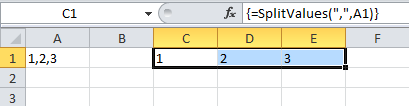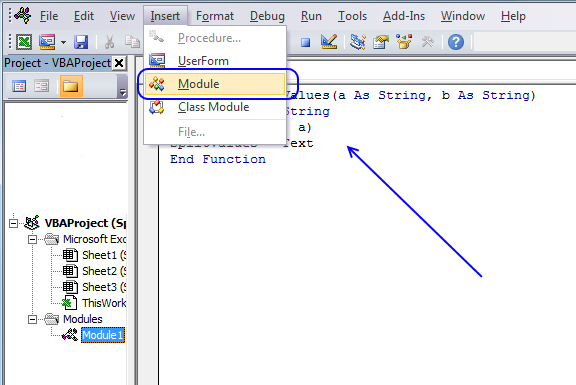Author: Oscar Cronquist Article last updated on July 11, 2018

The "Text to columns" feature in excel lets you split multiple values in a single cell using a delimiting character, across columns.

Where is this button?

1. Go to tab "Data" on the ribbon
2. Click "Text to columns" button

What happens if we record a macro while performing this action? This is what the macro recorder returns:

```Sub Macro1()
Selection.TextToColumns Destination:=Range("A1"), DataType:=xlDelimited, _
TextQualifier:=xlDoubleQuote, ConsecutiveDelimiter:=False, Tab:=False, _
Semicolon:=False, Comma:=True, Space:=False, Other:=False, FieldInfo _
:=Array(Array(1, 1), Array(2, 1), Array(3, 1)), TrailingMinusNumbers:=True
End Sub
```

The TextToColumns method and its parameters is explained here: Range.TextToColumns Method (Excel)

You an use the TextToColumns method in a macro but what about a user defined function? Unfortunately, you can´t use this method in a function. Don´t give up,  it is easy to build a basic udf that splits values across columns.

```Function SplitValues(a As String, b As String)
Dim Text() As String
Text = Split(b, a)
SplitValues = Text
End Function
```

### How to use it1. Select cell range C1:E1
2. Type =SplitValues(",",A1) in the formula bar
3. Press and hold CTRL + SHIFT
4. Press Enter

If you did this right, the formula has now a leading { and an ending }, like this {=SplitValues(",",A1)}. They appear automatically, don´t type them.

### Build a user defined function

To build a user defined function, follow these steps:

1. Press Alt + F11 to open the visual basic editor
2. Click "Insert" on the menu
3. Click "Module"4. Copy the code above and paste it to the code module.

### Explaining the user defined function

Function name and arguments

A user defined function procedure always start with "Function" and then a name. This udf has two arguments, a and b. Both a and b are strings.

```Function SplitValues(a As String, b As String)
```

Declaring variables

```Dim Text() As String
```

Text() is a dynamic string array. Read more about Defining data types.

Split function

```Text = Split(b, a)
```

The Split function accepts a text string and returns a zero-based, one-dimensional array containing all sub strings. Split allows you also to specify a delimiting character, default is the space character. The substrings are stored as multiple strings in Text array.

The udf returns the substrings in Text

```SplitValues = Text
```

End a udf

```End function
```

A function procedure ends with the "End function" statement.